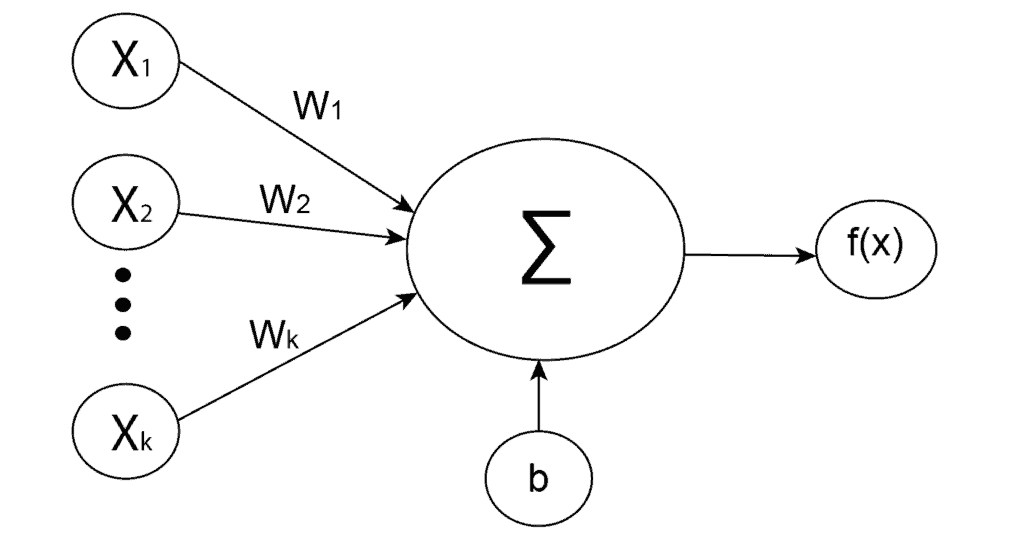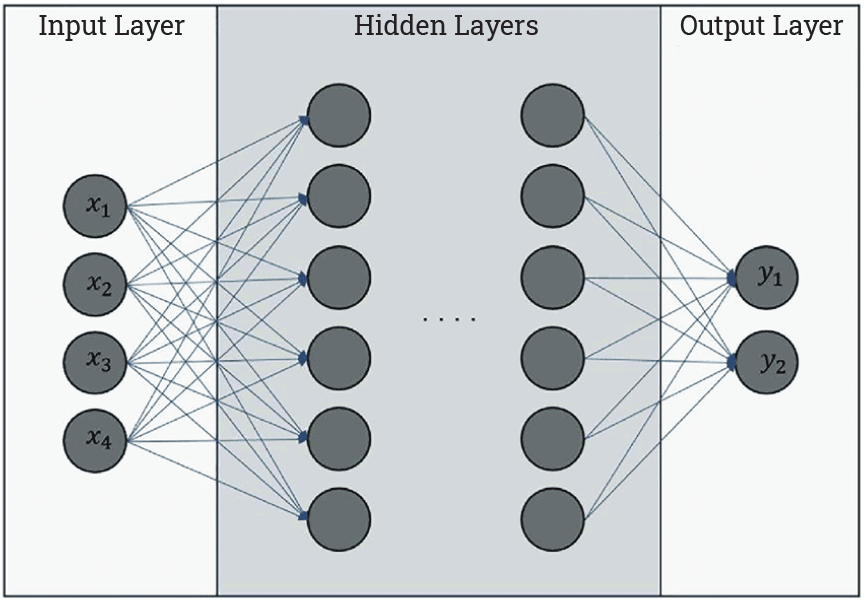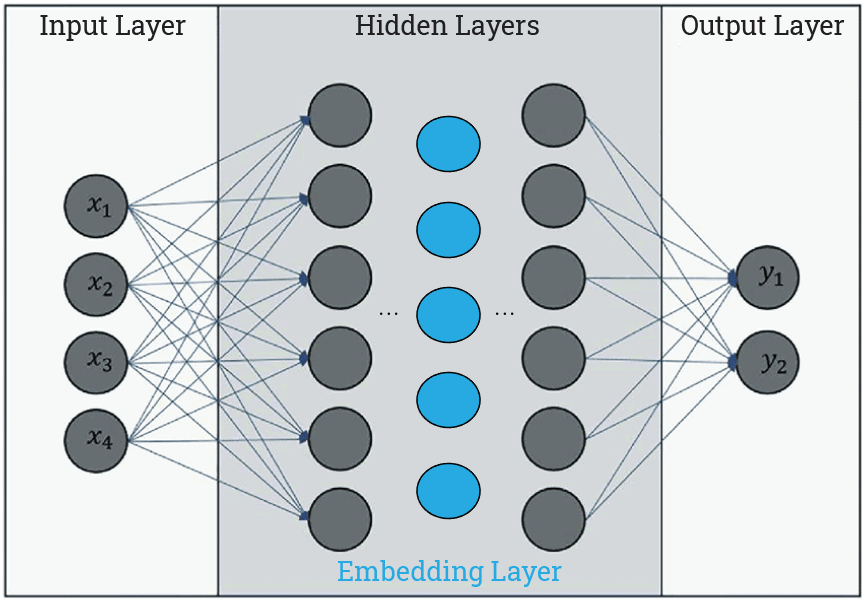## 1. Intro

In this tutorial, we’ll dive deep into the structure of neural networks. More precisely, we’ll explain what embedding layers in neural networks are and their applications.

To understand it better, firstly, we need to briefly explain what neural networks are and some of their components.

## 2. Neural Networks

Neural networks are a type of algorithm that mimics the structure and function of the human brain. They are designed to simulate biological neural networks and are made up of interconnected “neurons”. In general, the goal of neural networks is to create an artificial system that can process and analyze data in a similar way to the human brain works.

There are various types of neural networks, each with specific characteristics and applications. Broadly, we can divide them into three classes:

The main difference between them is the type of neurons that make them up and how information flows through the network.

## 3. Components of Neural Networks

As we mentioned above, there are many types of neural networks, each with specific characteristics and components. Despite that, some elements of neural networks are common for most of them.

### 3.1. Artificial Neurons

Artificial neurons are the basic building blocks of a neural network. They are units modelled after biological neurons. Each artificial neuron receives inputs and produces a single output, which we send to a network of other neurons.

Inputs are usually numeric values from a sample of external data, but they can also be other neurons’ outputs. Basically, a neuron receives an input value, performs a simple calculation on it, and transmits the result to the neurons ahead.

Mathematically, the simplest way of describing an artificial neuron is using the weighted sum:

(1)whereare weights,are inputs andbias. After that, an activation functionis applied to the weighted sum, which represents the final output of the neuron:### 3.2. Layers

In a neural network, a layer is a set of neurons that perform a specific task. Neural networks have multiple layers of interconnected neurons, and each layer performs a particular function.

Based on the position in a neural network, there are three types of layers:

• Input layer – responsible for receiving input data and passing it on to the next layer. This is the first layer in a neural network
• Hidden layers – can be found in almost every type of neural network except some single-layer types like perceptron. There can be multiple hidden layers in a neural network. The number of hidden layers and the number of neurons in each layer can vary depending on the complexity of the problem being solved
• Output layer – the last layer in a neural network which produces the final output or prediction

Here is a common graphical representation of them:## 4. Embedding Layers

An embedding layer is a type of hidden layer in a neural network. In one sentence, this layer maps input information from a high-dimensional to a lower-dimensional space, allowing the network to learn more about the relationship between inputs and to process the data more efficiently.

For example, in natural language processing (NLP), we often represent words and phrases as one-hot vectors, where each dimension corresponds to a different word in the vocabulary. These vectors are high-dimensional and sparse, which makes them difficult to work with.

Instead of using these high-dimensional vectors, the embedding layer could map each word to a low-dimensional vector, where each dimension represents a particular feature of the word:Theoretically, every hidden layer can represent an embedding layer. We can extract an output of any hidden layers and treat it as an embedding vector. Still, the point is not only to lower the input dimension but also to create a meaningful relationship between them.

That is why particular types of neural networks are used only to generate embeddings.

## 5. Types of Embedding Layers

The type of embedding layer depends on the neural network and the embedding process. There are several types of embedding that exist:

• Text embedding
• Image embedding
• Graph embedding and others

### 5.1. Text Embedding

Text embedding is probably the most common type of embedding. This is due to the popularity of transformers and language models.

One example of a language model is ChatGPT, made by the OpenAI team. Briefly, it has GPT-3 architecture and consists of two main components: an encoder and a decoder. Of course, the encoder part transforms an input text into an embedding vector, which the decoder part uses to generate an answer and convert it back to a text output.

While GPT-3 is a transformer model with both encoder and decoder parts, a more appropriate transformer model for embedding tasks with only an encoder part is BERT. It is a pre-trained model that encodes the language representation, such as characters, words, or sentences, so we can use embedding vectors for other tasks.

It’s important to mention that transformer-based models create contextual embeddings. It means that the same word will most likely get a different embedding vector if it appears in a different context. In opposite to that, there are some popular non-contextual architectures, such as word2vec and GloVe.

### 5.2. Image Embedding

Image embedding is a technique for representing images as dense embedding vectors. These vectors capture some visual features of the image, and we can use them for tasks such as image classification, object detection, and similar.

There are some popular pre-trained convolutional neural networks (CNN) that we can use to generate image embeddings. Some of them are NFNets, EfficientNets, ResNets, and others. In addition to CNNs, visual transformer models have recently gained popularity.

### 5.3. Graph Embedding

Analogously as for images, we use graph embedding to represent graphs as dense embedding vectors in a lower-dimensional space. Additionally, graph embedding is often related to node embedding, where the goal is to create an embedding vector for each individual node rather than for the entire graph.

Some of the popular algorithms for graph embeddings are:

• Locally Linear Embedding (LLE)
• DeepWalk
• Node2vec and others

## 6. Conclusion

In this article, we outlined the basics of neural networks, artificial neurons, and layers in neural networks. The main goal was to explain the purpose of embedding layers in neural networks. Also, we explained some of the applications of embeddings.

In summary, we use embedding layers in neural networks to convert input information into low-dimensional vector space, where each vector component is a specific input feature.